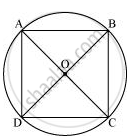# If Diagonals of a Cyclic Quadrilateral Are Diameters of the Circle Through the Vertices of the Quadrilateral, Prove that It is a Rectangle. - Mathematics

If diagonals of a cyclic quadrilateral are diameters of the circle through the vertices of the quadrilateral, prove that it is a rectangle.

#### SolutionLet ABCD be a cyclic quadrilateral having diagonals BD and AC, intersecting each other at point O.

angleBAD=1/2angleBOD=180^@/2=90^@              (Consider BD as a chord)

∠BCD = 180° − 90° = 90°

angleADC=1/2angleAOC=1/2(180^@) = 90^@            (Considering AC as a chord)

90° + ∠ABC = 180°

∠ABC = 90°

Each interior angle of a cyclic quadrilateral is of 90°. Hence, it is a rectangle.

Is there an error in this question or solution?
Chapter 10: Circles - Exercise 10.5 [Page 185]

#### APPEARS IN

NCERT Class 9 Maths
Chapter 10 Circles
Exercise 10.5 | Q 7 | Page 185

Share